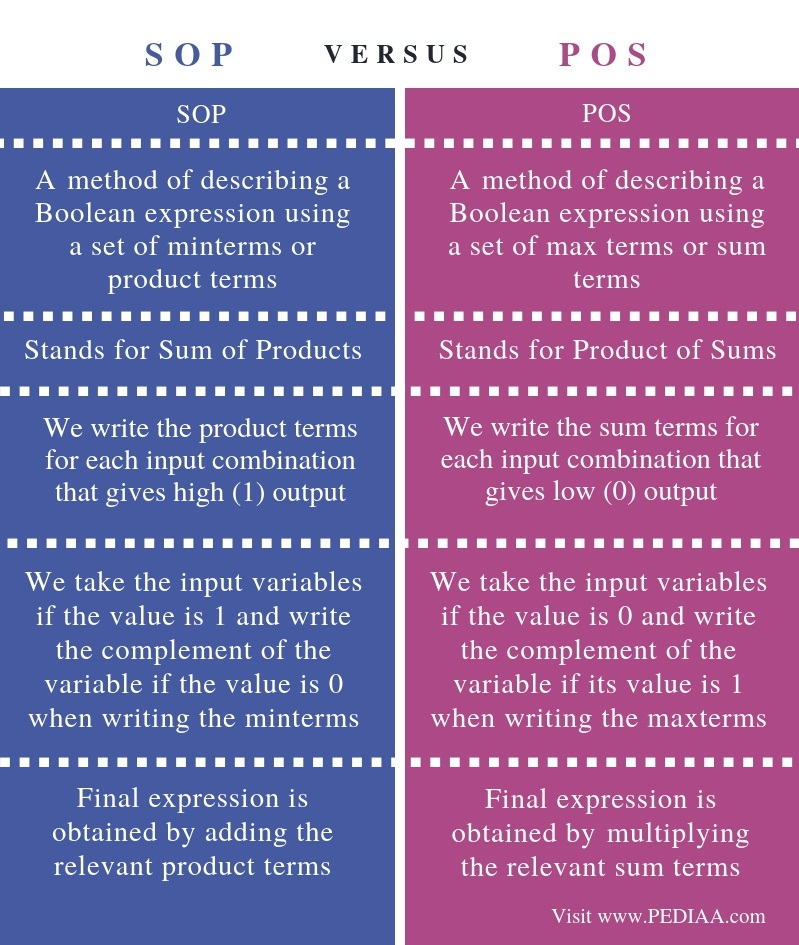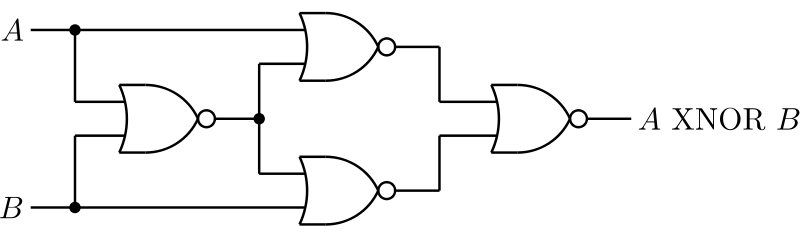# What is the Difference Between SOP and POS

The main difference between SOP and POS is that the SOP is a way of representing a Boolean expression using min terms or product terms while the POS is a way of representing a Boolean expression using max terms or sum terms.

Digital circuits use digital signals to operate. These signals have binary values; they can be either one or zero. Zero represents false or low state whereas one represents true or high state. Boolean algebra helps to describe the binary numbers and binary variables. To be more specific, a Boolean function is an algebraic form of Boolean expression. It is also possible to simplify Boolean functions of digital circuits using Boolean laws and theorems. Furthermore, SOP and POS are two methods of representing Boolean expressions.

### Key Areas Covered

1. What is SOP
– Definition, Functionality
2. What is POS
– Definition, Functionality
3. What is the Difference Between SOP and POS
– Comparison of Key Differences

### Key Terms

Boolean Function, SOP, POS## What is SOP

SOP stands for Sum of Products. Writing a Boolean expression using product terms is called Sum of Products form. The product terms are also known as min-terms. An example is as follows.Figure 1: Digital Circuit

For instance, assume that P and Q are input variables and F is the output variable. We take the complement of the variable for 0 and take the variable for 1. Then we can write the minterms by writing the product terms.

 P Q F Minterms 0 0 0 P’Q’ 0 1 1 P’Q 1 0 1 PQ’ 1 1 1 PQ

Finally, we can take the sum of all the minterms that has 1 for F. Therefore; the final expression is as follows.

F = P’Q + PQ’ + PQ

## What is POS

POS stands for Product of Sums. Writing a Boolean expression using sum terms is called Product of Sum form. We also call the sum terms as max-terms.

For example, assume that P and Q are input variables and F is the output variable. Here, we take the variable for 0 and take the complement of the variable for 1. Then we can write the max terms by writing the sum terms.

 P Q F Maxterms 0 0 0 P + Q 0 1 1 P+ Q’ 1 0 1 P’+Q 1 1 0 P’+Q’

Finally, we can take the product of all max terms that has 0 for F. Thus; the final expression is as follows.

F = (P+Q). (P’ + Q’)

## Difference Between SOP and POS

### Definition

SOP is a method of describing a Boolean expression using a set of minterms or product terms. POS is a method of describing a Boolean expression using a set of max terms or sum terms. Hence, these definitions themselves contain the main difference between SOP and POS.

### Long Form

Also, SOP stands for Sum of Products while POS stands for Product of Sums.

### Main Concern

To be more specific, the difference between SOP and POS is that, in SOP, we write the product terms for each input combination that gives high (1) output. Conversely, in POS, we write the sum terms for each input combination that gives low (0) output.

### Writing the Terms

Moreover, another difference between SOP and POS is that, in SOP, when writing the min terms, we take the input variables if the value is 1, but write the complement of the variable if the value is 0. However, in POS, when writing the max terms, we take the input variables if the value is 0, but write the complement of the variable if its value is 1.

### Output

One other difference between SOP and POS is that adding the relevant product terms gives the final expression in SOP while multiplying the relevant sum terms gives the final expression in POS.

### Conclusion

SOP and POS are two methods of representing Boolean expressions. The main difference between SOP and POS is that SOP is a way of representing a Boolean expression using min terms or product terms while POS is a way of representing a Boolean expression using max terms or sum terms.

##### Reference:

1. Suman, et al. “Boolean Functions(SOP, POS Forms).” Electronics Hub, 24 Dec. 2017, Available here.

##### Image Courtesy:

1. “XNOR using NOR” By Robert A. Maxwell – Own work (CC BY-SA 3.0) via Commons Wikimedia